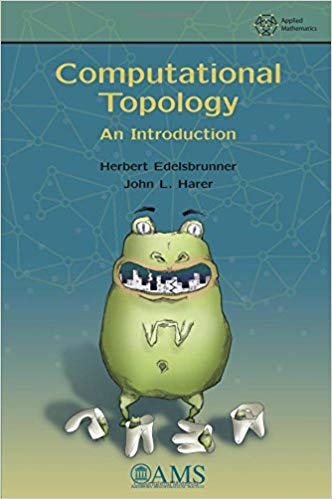Topological data analysis (TDA) is a technique in data science using topological methods to discern large-scale features. It complements classic techniques and adds insights other methods cannot detect. Connected components, holes and loops are typical features topological methods can help discover. For example, much like PCA indicates main directions of variance, loops can indicate periods in data. Topology is however much more than just visuals and algebraic methods bring along a solid stack of mathematics.

Persistent homology is often used synonymously with TDA and one can summarize it as

Persistent homology is an algebraic method for discerning topological features in data.

Let’s consider a set of data points (aka point cloud) like below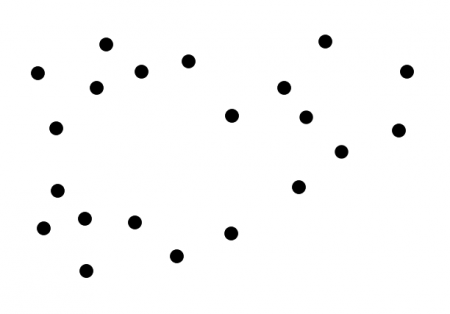If one draws circles with the points at the center, some of them will overlap and when they do we connect them like so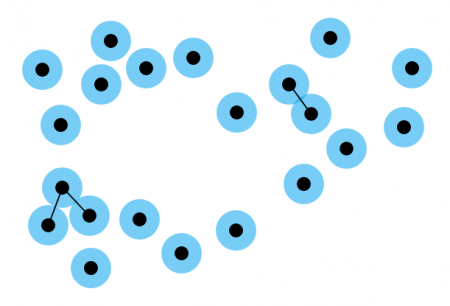If the radius is increased one gets three circles overlapping and this gives a triangle: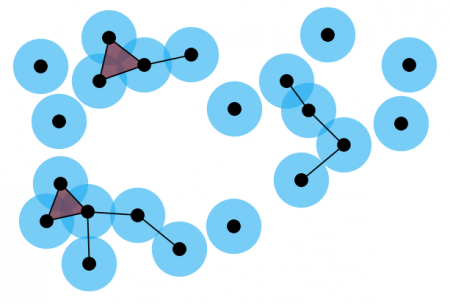Continuing in this fashion you eventually discover that there are two holes in the dataset. Furthermore, one gets higher dimensional ‘triangles’ called simplices. A 0-simplex is a point, a 1-simplex is a line and so on. A simplex is a collection of n-simplices and this is where simplicial homology starts (together with cohomology and lots of geometrical constructs).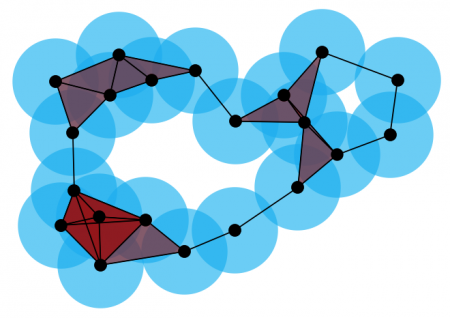If you continue increasing the radius you can see that the smaller hole disappears and eventually the bigger one as well.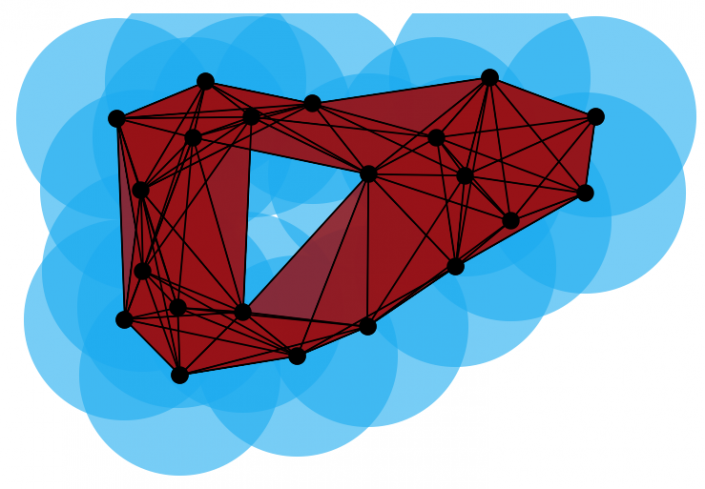It’s in general not possible to figure out what the correct radius is corresponding to a particular topological state. Instead, one looks at the whole range and marks the points where holes start to appear and where they stop to exist. This leads to the barcode representing the topological transitions. Below you can see how a bar is drawn for each 2-simplex and the bar ends when the simplex transitions to a 3-simplex (or higher). One can also draw bars for higher order simplices. The general idea with this representation is that the lasting bars represent genuine topological information while shorter ones are ‘noise’ (or transitional states).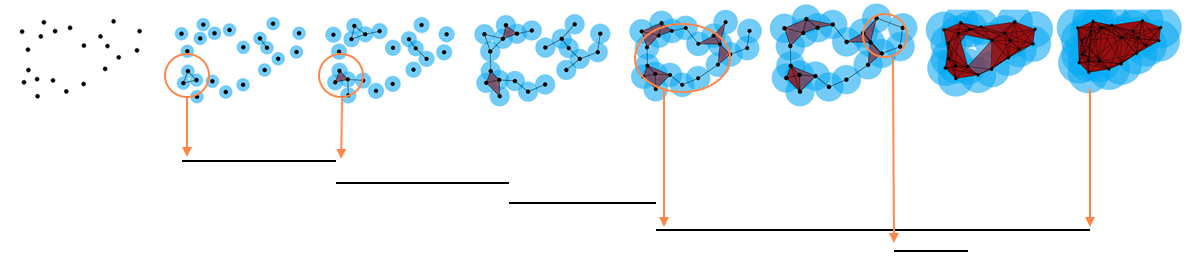All of this can be rigorously formulated and a good introduction to the subject is “Computational Topology” by Edelsbrunner and Harer.